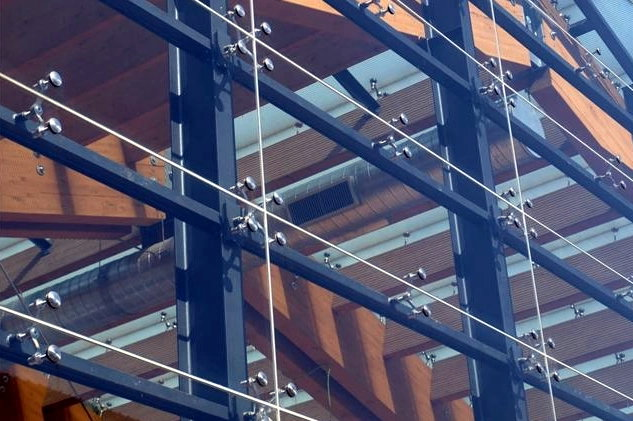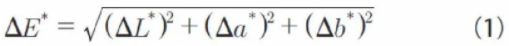#### 1. The detection principle

From 1931 to 1976, color scientists proposed various color difference formulas, such as ANLAB2, FMCH, and Hunter formulas, based on their respective fields and the actual data accumulated in this field. Different color-difference formulas cannot be converted to each other, making the calculation results of different formulas incomparable, and the results of many formulas are not satisfactory. This brings great inconvenience to practical industrial applications. To end this chaotic situation, it is necessary to unify the method of color difference evaluation. The International Commission on Illumination (CIE) compared and evaluated numerous color difference formulas, and recommended the CIE1964 unified color space and color difference formula and the 1976 CIELAB color space and its corresponding color difference formula in 1964 and 1976, respectively. application of the color difference formula (1).In the formula:

△E*——the color difference between two measured points;

△L*——the difference between the lightness index of the two measured points;

△a*/△b*——the difference between the chromaticity index of the two measured points.

In the CIELAB color space, the color difference tolerances of different regions are relatively close, and the position of the color sample in this space is represented by three-dimensional rectangular coordinates. The values of L*, a*, and b* can be calculated from the tristimulus values (X, Y, Z), where L* represents the lightness of the color, which represents the projected position of the color on the red-green axis, and b* represents the color Projected position in the direction of the yellow-blue axis.

Since the chromaticity system, CIELAB has greatly improved the inhomogeneity of the CIE1931 chromaticity system, it is feasible to use chromaticity to evaluate the color quality of products. The industry developed the initial international standards for color measurement and color difference evaluation based on the C1ELAB color difference formula. my country has also formulated various industry standards corresponding to it according to international standards. In the field of architectural glass, according to the international industry standard "Coated Glass", the color uniformity of coated glass is expressed by the color difference of CIELAB uniform color space, which should be ≤ 2.5. From the perspective of space, that is, the straight-line distance between two detection points in the space coordinate system (with L*, a*, b* as coordinate values) ≤ 2.5. The three-dimensional space model of the Lab (color model) color space is shown in Figure 1.

Figure 1 Three-dimensional space model diagram of Lab color space

#### 2. The test and analysis

From the space model diagram and the color difference formula, it can be found that the color difference between any two points on the glass surface is the linear distance between two coordinates in the color space. According to the requirements of the national standard, if the distance value is ≤2.5, it meets the standard requirements. However, in the actual visual perception, it is often found that even if the calculation result of the color difference formula is <2.5, the glass sample still has a relatively obvious color difference. Therefore, this study hypothesizes that there may be other unconsidered factors that have affected the judgment of color uniformity. To further clarify the causes of the above phenomena, it is necessary to test the actual curtain wall building and conduct data analysis and research from a practical point of view. This research takes a curtain wall building as the object. The facade of the building is a glass curtain wall structure, and the glass structure is 6 mm + 1.52 PVB + 6 (Double Silver Low-E Glass) + 8 mm. Observed from the outer surface, there is a visible color difference on the surface of the building, and it is mainly concentrated in the right half and lower half of the glass, which deviates from expectations to a certain extent. To verify the hypothesis of this research, a total of 112 glass samples were selected for the glass curtain wall construction project (including standard samples, which were confirmed by all parties involved in the project, and the color of the standard samples complied with the color standards stipulated in the architectural design scheme), the color uniformity test was carried out, and the lightness and chromaticity index of each test point were analyzed.

2.1 Standard sample color uniformity

According to the method stipulated in the national standard, a total of 112 pieces of curtain wall glass (including standard samples) were tested. For 565 testing points (take points according to the test method on the surface of each sample, it is stipulated that the upper left corner of the sample is the No. 1 testing point, the upper right corner is the No. 2 testing point, the center point is No. 3 testing point, and the lower left corner is No. 4 testing point. The color difference value of a point is the linear distance between two coordinates in the color space. According to the requirements of the national standard, the distance value ≤2.5 meets the standard requirements, but in the actual visual sense, it is often found that even the calculation result of the color difference formula is ＜2. 5. The glass sample still has an obvious color difference. Therefore, this study assumes that there may be other unconsidered factors that have affected the judgment of color uniformity.

To further understand the reasons for the above phenomenon, it is necessary to test the actual curtain wall building, to conduct data analysis and research from a practical point of view. This study takes a certain curtain wall building as the object. The facade of the building is a glass curtain wall structure, and the glass structure is 6 mm + 1.52 PVB + 6 (Double Silver Low-E Glass) + 8 mm. From the outside Observation from the surface shows that there is a visible color difference on the surface of the building, which is mainly concentrated in the right half and the lower half of the glass, and there is a certain deviation from the expectation. To verify the hypothesis of this study, a total of 112 glass curtain wall construction projects were selected. The glass samples (including standard samples, which have been jointly confirmed by all parties involved in the project, and the color of the standard samples conform to the color standards stipulated in the architectural design scheme) were tested for the color uniformity, and the lightness, Chromaticity index.

Figure 2 The distribution of chromatic aberration (△£*) of detection point No. 1

Figure 3 The lightness index of each detection point of the standard sample（L*）

Figure 4 The chromaticity index (a*) distribution of each test point of the standard sample

Figure 5 The chromaticity index (b*) distribution of each test point of the standard sample

2.2 Test the color uniformity of the sample

By detecting the lightness index and chromaticity index of each detection point on the surface of 111 detection templates, compare them with the standard template, and draw the corresponding atlas. Take the distribution of No. 1 and No. 4 detection point atlases as an example, see Fig. 6 ~ Fig. 13.

Figure 6 The color difference (△E*) distribution of No. 1 detection point

Figure 7 The lightness index (L*) distribution of No. 1 detection point

Figure 8 The chromaticity index (a*) distribution of No. 1 detection point

Figure 9 The chromaticity index (b*) distribution of No. 1 detection point

Figure 10 The color difference (△E*) distribution of No. 4 detection point

Figure 11 The lightness index (L*) distribution of No. 4 detection point

Figure 12 The chromaticity index (a*) distribution of No. 4 detection point

Figure 13 The chromaticity index (b*) distribution of No. 4 detection point

It can be seen from the comparison spectrum of each test point that the color difference (AE*) of most test sample test points is within the range specified by the standard, but further observation of lightness and chromaticity index, it will be found that the lightness value of the corresponding position of the sample is generally Above the value on the standard, the chromaticity index (a*) coordinates are on the negative axis and the chromaticity index (b*) coordinates are on the positive axis. And from the spectrum distribution of No. 1 and No. 4 detection points, the chromaticity index (b*) itself is unstable. Therefore, the color difference (AE*) in the test results generally meets the specified requirements, but the color of the corresponding test point will be green and yellow compared with the standard sample, and there are still differences in visual perception.

Through further analysis of the color difference (AE*) distribution, the areas where the color difference is relatively concentrated in each test sample are No. 2, 4, and 5 test points. Among them, No. 2 and No. 5 test points are located in the same side area of the test sample, and both are in the right area; No. 4 and No. 5 test points are located in the same side area of the test sample, and both are in the lower area. At the same time, comparing the color difference (AE*) between each test inside the single test sample, it was found that the problem of color difference (AE*) between the No. 2 test point and No. 3, 4, and 5 test points is relatively concentrated.The above situation is consistent with the intuitive visual color difference of the facade. Therefore, the study believes that for the color difference detection of architectural glass, not only the color difference results but also the lightness and chromaticity index should be considered. Although lightness and chromaticity index are process quantities, their deviation directly reflects the color difference between the detection points.

#### 3 The considerations on the color uniformity detection of architectural glass

According to the test results of the actual project, it can be confirmed that the test data (color difference AE*) obtained according to the international standard has basically verified the difference in the actual visual sense, but for the case of the color difference AE* value ≤2.5, lightness and chromaticity should be considered The absolute value deviation of the index. From a spatial point of view, even if the distance between two color space coordinate points is within the specified range, it may still cause obvious visual color deviation if there is a large one-way deviation in the lightness or chromaticity index coordinates.

In the development of urban construction, building curtain walls occupy a considerable proportion, and glass curtain walls are the main form. Therefore, the color uniformity of the appearance of building glass is of great significance to the effect of single buildings and regional planning. Therefore, regarding the test method for the color uniformity of architectural glass, it is suggested that on the basis of the test method stipulated in the current national standards, in addition to the linear distance of the space coordinate system of the two test points, the simultaneous increase of the lightness AL* and chromaticity index Aa*, the consideration of the absolute difference of Ab* (for example: increase the upper limit of the absolute value of the difference of AL*, Aa*, Ab* between two color space coordinate points), reduce the individual cases, although the calculation result of the color difference value meets the requirements, but the extreme difference in lightness and chromaticity index still affects the actual visual sense.